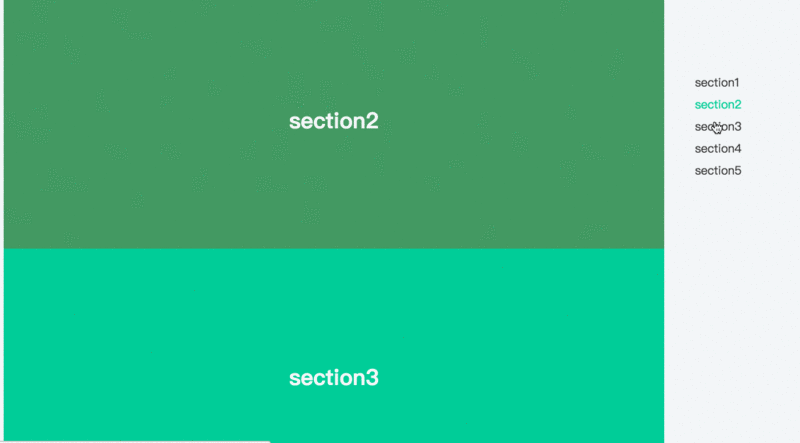# Javascript实现页面滚动时导航智能定位

更新时间：2017年05月06日 10:23:29   作者：wangkun

```<div class="container">
<div class="wrapper">
<div class="section" id="section1">section1</div>
<div class="section" id="section2">section2</div>
<div class="section" id="section3">section3</div>
<div class="section" id="section4">section4</div>
<div class="section" id="section5">section5</div>
</div>
<nav>
<a href="#section1" rel="external nofollow" class="current">section1</a>
<a href="#section2" rel="external nofollow" >section2</a>
<a href="#section3" rel="external nofollow" >section3</a>
<a href="#section4" rel="external nofollow" >section4</a>
<a href="#section5" rel="external nofollow" >section5</a>
</nav>
</div>
```

js代码如下：

```var \$navs = \$('nav a'),          // 导航
\$sections = \$('.section'),       // 模块
\$window = \$(window),
navLength = \$navs.length - 1;

\$window.on('scroll', function() {
var scrollTop = \$window.scrollTop(),
len = navLength;

for (; len > -1; len--) {
var that = \$sections.eq(len);
if (scrollTop >= that.offset().top) {
break;
}
}
});

``````\$navs.on('click', function(e) {
e.preventDefault();
\$('html, body').animate({
'scrollTop': \$(\$(this).attr('href')).offset().top
}, 400);
});
```